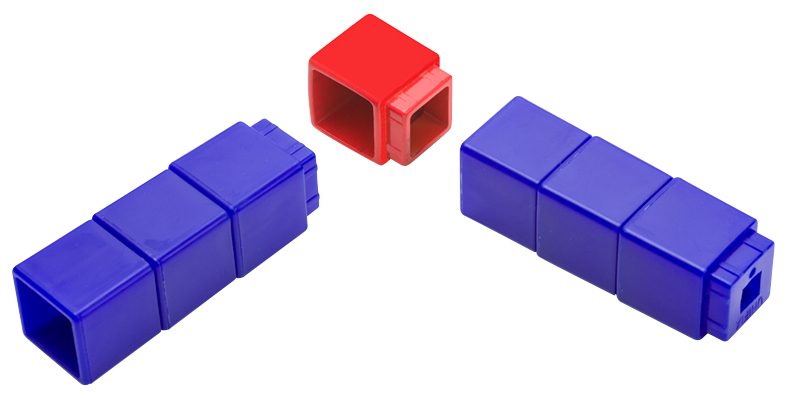The first time I ever saw a teacher using Unifix cubes in the classroom, it was not in an elementary school. I was coaching a middle school teacher who was introducing the concepts of mean, median, and mode to her students. They were using the cubes to “graph” the data and then find these measures of central tendency. Since then, I’ve seen Unifix cubes in classrooms at every grade level, used in a variety of ways to teach number sense, data, measurement, patterns, and an array of topics. They were never as useful a tool for Geometry… until now.

A simple new Unifix cube allows us to turn the corner with this familiar manipulative: the Corner Cube. No longer limited to straight lines, students can now explore geometric concepts. Here are a couple of ideas to help you get started:## Square or Rectangle?

Give each student four corner cubes and several regular Unifix cubes. Have each student build a shape with four sides. As a class or in small groups, have students classify their shape as a rectangle or a square, explaining how they know. This simple, hands-on activity reinforces the similarities and differences between rectangles and squares. Extension: Challenge students to change their rectangle into a square or vice-versa.

## How Far Around?

Give each student four corner cubes and several regular Unifix cubes. Have each student build a rectangle (or square) and count the number of cubes it takes to go around. Ask students if they can find a different rectangle that uses the same number of cubes. Extension: Have students calculate the number of cubes “inside” their shape, and notice how this number changes with a different shape. This activity introduces the ideas of perimeter and area in a very informal way.

Corner Cubes allow students to use a familiar manipulative in a new way to explore math concepts. As they work with shapes and measurement in two dimensions, students’ curiosity is rewarded and they are better able to make the jump to abstract representations.